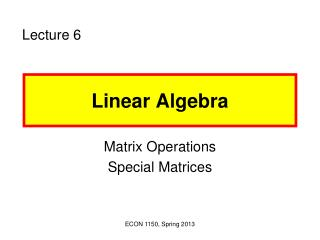DownloadDownload PresentationLinear Algebra

# Linear Algebra

Download Presentation## Linear Algebra

- - - - - - - - - - - - - - - - - - - - - - - - - - - E N D - - - - - - - - - - - - - - - - - - - - - - - - - - -
##### Presentation Transcript

1. Lecture 6 Linear Algebra Matrix Operations Special Matrices ECON 1150, Spring 2013

2. 1. Systems of Linear Equations Consider the following equations: x1 + 2x2 = 2 (1) 2x1 – x2 = 4 (2) Method of substitution Method of elimination Solution: (x1*, x2*) ECON 1150, Spring 2013

3. Consider the following equations: 4x1 – x2 + 2x3 = 13 (1) x1 + 2x2 – 2x3 = 0 (2) -x1 + x2 + x3 = 5 (3) How is the solution (x1*, x2*, x3*) found? ECON 1150, Spring 2013

4. Row operations: • Multiply an equation by a nonzero constant • Add a multiple of one equation to another • Interchange any two equations Gaussian Elimination x1* = 2, x2* = 3, x3* = 4 ECON 1150, Spring 2013

5. General rule: To solve a system of linear equations with a unique solution, the number of linearly independent equations must be equal to the number of variables. If any equation of a system of equations can be derived from a series of row operations on that system, then this system is called linearly dependent. Otherwise, it is called linearly independent. ECON 1150, Spring 2013

6. The following systems are linearly dependent. 2x + y = 8 (1) x – y = 1 (2) -1.5x + 3y = 1.5 (3) – x – y + z = –2 (4) 3x + 2y – 2z = 7 (5) x –y +z = 4 (6) ECON 1150, Spring 2013

7. Equation system: m linearly independent equations n unknowns Case 1: m = n  unique solution x + y = 10, x – y = 6 Case 2: m < n  Infinitely many solutions x + y = 10 Case 3: m > n  No solution. x + y = 10, x – y = 4, 2x – 3y = 6 ECON 1150, Spring 2013

8. General equation system • Matrix algebra can help to • simplify the expression • solve the system efficiently • testing the existence of a solution ECON 1150, Spring 2013

9. = 2. Matrices and Vectors A matrix is a rectangular array of numbers. ECON 1150, Spring 2013

10. 2x2 2x3 Order (dimension) of a matrix = (# of rows) x (# of columns) Row vector: a matrix with only one row Column vector: a matrix with only one column [3 5 -6 1] ECON 1150, Spring 2013

11. 2x2 2x2 Two matrices are equal if they have the same order and the corresponding elements are equal. E.g., x = y = 2. ECON 1150, Spring 2013

12. 3. Matrix Operations Addition and subtraction Adding up elements of the same corresponding position Conformability: Same order Example6.1: ECON 1150, Spring 2013

13. Scalar multiplication Conformability: Not required ECON 1150, Spring 2013

14. Multiplication Conformability: The number of the columns of A is equal to the number of rows of B. Rule of matrix multiplication: The element of the ith row and jth column of matrix C ith row of Ajthcolumn of B and adding the resulting products. ECON 1150, Spring 2013

15. 1x1 1x3 3x1 1x2 1x3 3x2 ECON 1150, Spring 2013

16. 2x3 2x1 3x1 ECON 1150, Spring 2013

17. Let C = AB = . Then c1 = 4(7) + 11(2) c2 = 17(7) + 6(2) ECON 1150, Spring 2013

18. ECON 1150, Spring 2013

19. Remark: • (AB)C = A(BC) • A(B + C) = AB + AC • (A + B)C = AC + BC ECON 1150, Spring 2013

20. 4. Special Matrices 4.1 Square Matrices # of rows = # of columns E.g., ECON 1150, Spring 2013

21. 4.2 Identity Matrices For any M by N matrix A, IMA = AIN = A. 4.3 Null Matrices A matrix whose elements are all zero. ECON 1150, Spring 2013

22. 4.4 The transpose of a matrix A is a new matrix AT such that the ith row of A is the ith column of AT. 4.5Symmetric matrices A square matrix that is equal to its transpose. ECON 1150, Spring 2013